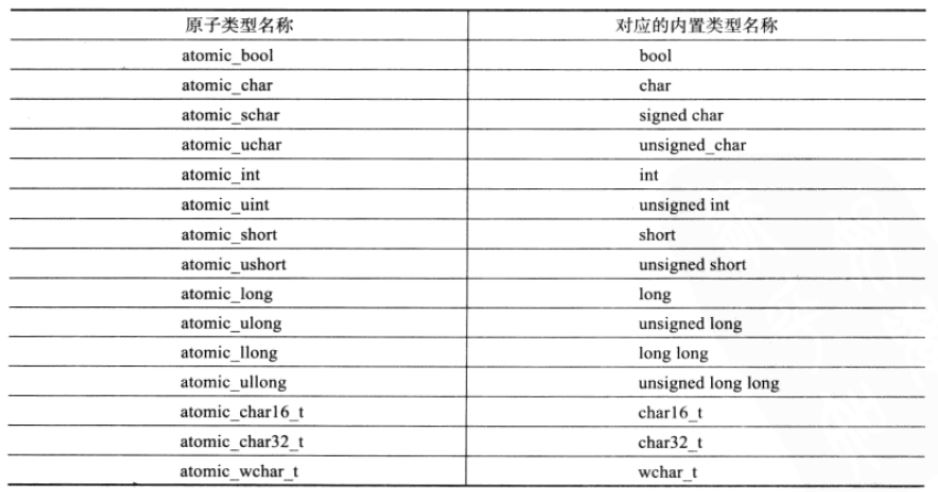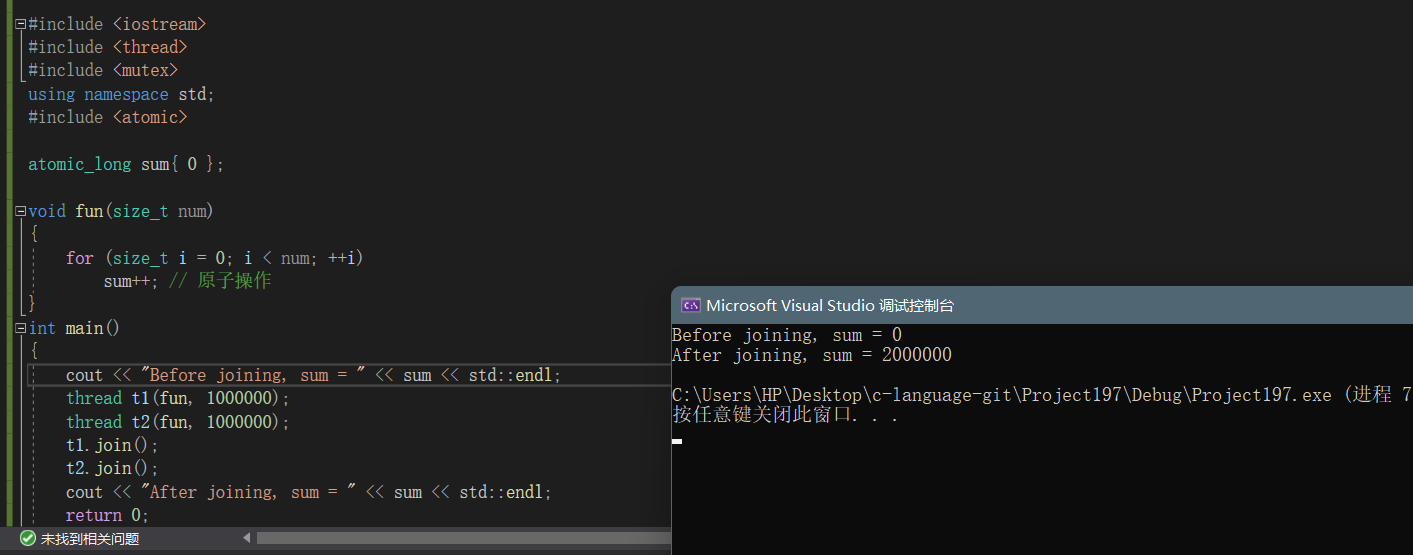﻿ 深入解析C++11 lambda表达式/包装器/线程库_C 语言_脚本之家
C 语言# 深入解析C++11 lambda表达式/包装器/线程库

## 一、lambda表达式

### 1、lambda的引入

```#include <algorithm>
#include <functional>
int main()
{
int array[] = { 4,1,8,5,3,7,0,9,2,6 };
// 默认按照小于比较，排出来结果是升序
std::sort(array, array + sizeof(array) / sizeof(array));
for (int i = 0; i < 10; i++)
{
cout << array[i] << " ";
}cout << endl;
// 如果需要降序，需要改变元素的比较规则
std::sort(array, array + sizeof(array) / sizeof(array), greater<int>());
for (int i = 0; i < 10; i++)
{
cout << array[i] << " ";
}cout << endl;
return 0;
}``````struct Goods
{
string _name;
double _price;
};
struct Compare
{
bool operator()(const Goods& gl, const Goods& gr)
{
return gl._price <= gr._price;
}
};
int main()
{
Goods gds[] = { { "苹果", 2.1 }, { "香蕉", 3 }, { "橙子", 2.2 }, {"菠萝", 1.5} };
sort(gds, gds + sizeof(gds) / sizeof(gds), Compare());
for (int i = 0; i < 4; i++)
cout << gds[i]._name << ":"<<gds[i]._price<<" ";
cout << endl;
return 0;
}``````int main()
{
Goods gds[] = { { "苹果", 2.1 }, { "香蕉", 3 }, { "橙子", 2.2 }, {"菠萝", 1.5} };
sort(gds, gds + sizeof(gds) / sizeof(gds), [](const Goods& l, const Goods& r)
->bool
{
return l._price < r._price;
});
return 0;
}```

### 2、lambda表达式语法

lambda表达式书写格式：

`[capture-list] (parameters) mutable -> return-type { statement }`

lambda表达式各部分说明：

[capture-list] :

(parameters)：

mutable：

->returntype：

{statement}：

```int main()
{
// 最简单的lambda表达式, 该lambda表达式没有任何意义
[] {};
// 省略参数列表和返回值类型，返回值类型由编译器推导为int
int a = 3, b = 4;
[=]{ return a + 3; };
// 省略了返回值类型，无返回值类型
auto fun1 = [&](int c) { b = a + c; };
fun1(10);
cout << a << " " << b << endl;
// 各部分都很完善的lambda函数
auto fun2 = [=, &b](int c)->int {return b += a + c; };
cout << fun2(10) << endl;
// 复制捕捉x
int x = 10;
auto add_x = [x](int a)mutable { x *= 2; return a + x; };//传值捕捉修改需要mutable修饰
auto add_x1 = [&x](int a){ x *= 2; return a + x; };//引用捕捉不用
cout << x << endl;
cout << x << endl;
return 0;
}
```### 3、捕获列表说明

```[var]：表示值传递方式捕捉变量var
[=]：表示值传递方式捕获所有父作用域中的变量(包括this)
[&var]：表示引用传递捕捉变量var
[&]：表示引用传递捕捉所有父作用域中的变量(包括this)
[this]：表示值传递方式捕捉当前的this指针```

lambda表达式之间不能相互赋值，即使看起来类型相同

```void (*PF)();
int main()
{
auto f1 = []{cout << "hello world" << endl; };
auto f2 = []{cout << "hello world" << endl; };
//f1 = f2; // 编译失败--->提示找不到operator=()
// 允许使用一个lambda表达式拷贝构造一个新的副本
auto f3(f2);
f3();
// 可以将没有捕获任何变量的lambda表达式赋值给相同类型的函数指针
PF = f2;
PF();
return 0;
}
```

Lambda是实现了函数调用运算符的匿名类(anonymous class)。对于每一个Lambda，编译器创建匿名类，并定义相应的数据成员存储Lambda捕获的变量。没有捕获变量的Lambda不包含任何含成员变量。一个没有任何成员变量(包括没有虚函数表指针)的类型，在空指针上调用成员函数也不会有任何的问题，因为它的成员函数不会通过this指针访问内存。当Lambda向函数指针的转换时，编译器为Lambda的匿名类实现函数指针类型转换运算符

### 4、函数对象与lambda表达式

```class Rate
{
public:
Rate(double rate): _rate(rate)
{}
double operator()(double money, int year)
{
return money * _rate * year;
}
private:
double _rate;
};
int main()
{
// 函数对象
double rate = 0.49;
Rate r1(rate);
r1(10000, 2);
// lamber
auto r2 = [=](double monty, int year)->double{return monty*rate*year; };
r2(10000, 2);
return 0;
}
```## 二、包装器

### 1、function包装器

function包装器也叫作适配器，C++中的function本质是一个类模板，也是一个包装器

```template<class F, class T>
T useF(F f, T x)
{
static int count = 0;
cout << "count:" << ++count << endl;
cout << "count:" << &count << endl;
return f(x);
}
double f(double i)
{
return i / 2;
}
struct Functor
{
double operator()(double d)
{
return d / 3;
}
};
int main()
{
// 函数名
cout << useF(f, 11.11) << endl;
// 函数对象
cout << useF(Functor(), 11.11) << endl;
// lamber表达式
cout << useF([](double d)->double { return d / 4; }, 11.11) << endl;
return 0;
}
``````// 类模板原型如下
template <class T> function; // undefined
template <class Ret, class... Args>
class function<Ret(Args...)>;
```

Ret: 被调用函数的返回类型

Args…：被调用函数的形参

```#include <functional>
int f(int a, int b)
{
return a + b;
}
struct Functor
{
int operator() (int a, int b)
{
return a + b;
}
};
class Plus
{
public:
static int plusi(int a, int b)
{
return a + b;
}
double plusd(double a, double b)
{
return a + b;
}
};
int main()
{
// 函数名(函数指针)
std::function<int(int, int)> func1 = f;
cout << func1(1, 2) << endl;
// 函数对象
std::function<int(int, int)> func2 = Functor();
cout << func2(1, 2) << endl;
// lamber表达式
std::function<int(int, int)> func3 = [](const int a, const int b){
return a + b;
};
cout << func3(1, 2) << endl;
// 类的成员函数
std::function<int(int, int)> func4 = Plus::plusi;
cout << func4(1, 2) << endl;
std::function<double(Plus, double, double)> func5 = &Plus::plusd;//对于普通成员的包装一定要加上&，需要通过指针进行调用成员函数
cout << func5(Plus(), 1.1, 2.2) << endl;//传入类对象，通过对象进行调用
return 0;
}
``````#include <functional>
template<class F, class T>
T useF(F f, T x)
{
static int count = 0;
cout << "count:" << ++count << endl;
cout << "count:" << &count << endl;
return f(x);
}
double f(double i)
{
return i / 2;
}
struct Functor
{
double operator()(double d)
{
return d / 3;
}
};
int main()
{
//将多个可调用类型进行封装成相同类型，便于统一调用
// 函数名
std::function<double(double)> func1 = f;
cout << useF(func1, 11.11) << endl;
// 函数对象
std::function<double(double)> func2 = Functor();
cout << useF(func2, 11.11) << endl;
// lamber表达式
std::function<double(double)> func3 = [](double d)->double {
return d /4;
};
cout << useF(func3, 11.11) << endl;
return 0;
}
```### 2、bind 概念：

std::bind函数定义在头文件中，是一个函数模板，它就像一个函数包装器(适配器)，接受一个可调用对象（callable object），生成一个新的可调用对象来“适应”原对象的参数列表一般而言，我们用它可以把一个原本接收N个参数的函数fn，通过绑定一些参数，返回一个接收M个（M可以大于N，但这么做没什么意义）参数的新函数；同时，使用std::bind函数还可以实现参数顺序调整等操作

```#include <functional>
int Plus(int a, int b)
{
return a + b;
}
class Sub
{
public:
int sub(int a, int b)
{
return a - b;
}
static int sub1(int a, int b)
{
return a - b;
}
};
int main()
{
//普通函数的绑定
//表示绑定函数plus 参数分别由调用 func1 的第一，二个参数指定(placeholders用来表示参数位占位)
std::function<int(int, int)> func1 = std::bind(Plus, placeholders::_1,placeholders::_2);
//auto func1 = std::bind(Plus, placeholders::_1, placeholders::_2);直接使用auto识别类型

//表示绑定函数 plus 的第一，二参数为： 1， 2
auto func2 = std::bind(Plus, 1, 2);
cout << func1(1, 2) << endl;
cout << func2(2,3) << endl;//func2();也可以不用传参数-因为参数已经绑定好了，传入的参数没有实际的作用

//类函数的绑定
//类的成员函数必须通过类的对象或者指针调用，因此在bind时，bind的第一个参数的位置来指定一个类的实列、指针或引用。
Sub s;
// 绑定成员函数
std::function<int(int, int)> func3 = std::bind(&Sub::sub, s,placeholders::_1, placeholders::_2);
// 参数调换顺序
std::function<int(int, int)> func4 = std::bind(&Sub::sub, s,placeholders::_2, placeholders::_1);
std::function<int(int, int)> func5 = std::bind(Sub::sub1,placeholders::_2, placeholders::_1);//静态成员函数的绑定-不需要类的示例指针或引用
cout << func3(1, 2) << endl;
cout << func4(1, 2) << endl;
cout << func5(1, 2) << endl;
return 0;
}
```bind是对包装的可调用类型的进一步封装，可以根据自己的需要进行调整参数的数据及位置，绑定类对象能有优化成员函数的包装使用，更加符合使用习惯

## 三、线程库

### 1、线程的概念及使用

get_id()获取线程id
jionable()线程是否还在执行，joinable代表的是一个正在执行中的线程。
jion()该函数调用后会阻塞住线程，当该线程结束后，主线程继续执行
detach()在创建线程对象后马上调用，用于把被创建线程与线程对象分离开，分离 的线程变为后台线程，创建的线程的"死活"就与主线程无关

```#include <thread>
int main()
{
cout << t1.get_id() << endl;
return 0;
}```

```// vs下查看
typedef struct
{ /* thread identifier for Win32 */
void *_Hnd; /* Win32 HANDLE */
unsigned int _Id;
} _Thrd_imp_t;```

1. 函数指针
2. lambda表达式
3. 函数对象

```#include <iostream>
using namespace std;
{
cout << "Thread1" << a << endl;
}
class TF
{
public:
void operator()()
{
}
};
int main()
{
// 线程函数为函数指针
// 线程函数为lambda表达式
});
// 线程函数为函数对象
TF tf;
t1.join();
t2.join();
t3.join();
cout << "Main thread!" << endl;
return 0;
}```### 2、线程函数参数

```#include <iostream>
using namespace std;
void Func1(int& x)
{
x += 10;
return;
}
void Func2(int* x)
{
*x += 10;
return;
}
int main()
{
int a = 10;
// 在线程函数中对a修改，不会影响外部实参，因为：线程函数参数虽然是引用方式，但其实际引用的是线程栈中的拷贝
// vs2019会报错-对于引用的参数这么传入
//t1.join();
//cout << a << endl;
// 如果想要通过形参改变外部实参时，必须借助std::ref()函数
t2.join();
cout << a << endl;
// 地址的拷贝
t3.join();
cout << a << endl;
return 0;
}
``````#include <iostream>
using namespace std;
class A
{
public:
void Func1(int x)
{
cout << x << endl;
}
static void Func2(int x)
{
cout << x << endl;
}
};
int main()
{
A a;
//普通成员函数需要传入类的实例或者指针
t1.join();
//静态成员函数则不用
t2.join();
return 0;
}
```### 3、原子性操作库(atomic)

```#include <iostream>
using namespace std;

unsigned long sum = 0L;
void fun(size_t num)
{
for (size_t i = 0; i < num; ++i)
sum++;
}
int main()
{
cout << "Before joining,sum = " << sum << std::endl;
t1.join();
t2.join();
cout << "After joining,sum = " << sum << std::endl;
return 0;
}
```C++98中传统的解决方式：可以对共享修改的数据可以加锁保护

```#include <iostream>
#include <mutex>
using namespace std;

std::mutex m;
unsigned long sum = 0L;
void fun(size_t num)
{
for (size_t i = 0; i < num; ++i)
{
m.lock();
sum++;
m.unlock();
}
}
int main()
{
cout << "Before joining,sum = " << sum << std::endl;
t1.join();
t2.join();
cout << "After joining,sum = " << sum << std::endl;
return 0;
}
``````#include <iostream>
using namespace std;
#include <atomic>

atomic_long sum{ 0 };
void fun(size_t num)
{
for (size_t i = 0; i < num; ++i)
sum++; // 原子操作
}
int main()
{
cout << "Before joining, sum = " << sum << std::endl;
t1.join();
t2.join();
cout << "After joining, sum = " << sum << std::endl;
return 0;
}
``````int main()
{
t1.join();
t2.join();
//printf("%d\n", sum);vs2019存在类型不匹配问题
//解决方式
//1.
//2.
cout << sum << endl;
//3.
printf("%ld\n", (long)sum);
return 0;
}
```

atomic类模板:

```atmoic<T> t; // 声明一个类型为T的原子类型变量t
```

```#include <atomic>
int main()
{
atomic<int> a1(0);
//atomic<int> a2(a1); // 编译失败
atomic<int> a2(0);
//a2 = a1; // 编译失败
return 0;
}```

### 4、lock_guard与unique_lock

#### 1、mutex的种类

C++11提供的最基本的互斥量，该类的对象之间不能拷贝，也不能进行移动

mutex最常用的三个函数：

lock()上锁：锁住互斥量
unlock()解锁：释放对互斥量的所有权
try_lock()尝试锁住互斥量，如果互斥量被其他线程占有，则当前线程也不会被阻塞

std::recursive_mutex

std::timed_mutex

try_lock_for()

try_lock_until()接受一个时间点作为参数，在指定时间点未到来之前线程如果没有获得锁则被阻塞住，如果在此期间其他线程释放了锁，则该线程可以获得对互斥量的锁，如果超时（即在指定时间内还是没有获得锁），则返回 false

std::recursive_timed_mutex

recursive_mutex和timed_mutex的结合

#### 2、lock_guard

```template<class _Mutex>
class lock_guard
{
public:
// 在构造lock_gard时上锁
explicit lock_guard(_Mutex& _Mtx)
: _MyMutex(_Mtx)
{
_MyMutex.lock();
}
: _MyMutex(_Mtx)
{}
// 在析构lock_gard时解锁
~lock_guard() _NOEXCEPT
{
_MyMutex.unlock();
}
lock_guard(const lock_guard&) = delete;
lock_guard& operator=(const lock_guard&) = delete;
private:
_Mutex& _MyMutex;
};
```

lock_guard类模板主要是通过RAII的方式，对其管理的互斥量进行了封装，在需要加锁的地方，只需要用上述介绍的任意互斥体实例化一个lock_guard，调用构造函数成功上锁，出作用域前，lock_guard对象要被销毁，调用析构函数自动解锁，可以有效避免死锁问题

lock_guard的缺陷：

### 5、两个线程交替打印奇数偶数

```#include <iostream>
#include <mutex>
using namespace std;
int main()
{
int i = 1;
int j = 2;
bool flg = true;
mutex mtx;
condition_variable cv;
//存在时间片切出去的问题
while (j <= 100)
{
std::unique_lock<mutex> lock(mtx);
cv.wait(lock);
cout << std::this_thread::get_id() << ":" << j << endl;
j += 2;
cv.notify_one();
}
});
while (i <= 100)
{
std::unique_lock<mutex> lock(mtx);
cout << std::this_thread::get_id() << ":" << i << endl;
i += 2;
cv.notify_one();
cv.wait(lock);
}
});
t1.join();
t2.join();
return 0;
}``````#include <iostream>
#include <mutex>
#include <condition_variable>
using namespace std;
int main()
{
int i = 1;
int j = 2;
bool flg = true;
mutex mtx;
condition_variable cv;
//正确写法
while (i <= 100)
{
std::unique_lock<mutex> lock(mtx);
cv.wait(lock, [&flg]() { return flg; });//根据条件判断是否需要进行阻塞等待
cout << std::this_thread::get_id() << ":" << i << endl;
i+=2;
flg = false;//更改条件变量-使得另一个线程执行，该线程会等待住
cv.notify_one();//进行唤醒等待条件变量下的线程
}
});
thread t2([&j, &mtx, &cv, &flg]() {
while (j <= 100)
{
std::unique_lock<mutex> lock(mtx);
cv.wait(lock, [&flg]() { return !flg; });
cout << std::this_thread::get_id() << ":" << j << endl;
j += 2;
flg = true;
cv.notify_one();
}
});
t1.join();
t2.join();
return 0;
}
``````#include <iostream>
#include <mutex>
#include <condition_variable>
using namespace std;
int main()
{
int i = 1;
int j = 2;
bool flg = true;
mutex mtx;
condition_variable cv;
//正确写法
while (i <= 100)
{
std::unique_lock<mutex> lock(mtx);
cv.wait(lock, [&flg]() { return flg; });//根据条件判断是否需要进行阻塞等待
cout << std::this_thread::get_id() << ":" << i << endl;
i+=2;
flg = false;//更改条件变量-使得另一个线程执行，该线程会等待住
cv.notify_one();//进行唤醒等待条件变量下的线程
}
});
thread t2([&j, &mtx, &cv, &flg]() {
while (j <= 100)
{
std::unique_lock<mutex> lock(mtx);
cv.wait(lock, [&flg]() { return !flg; });
cout << std::this_thread::get_id() << ":" << j << endl;
j += 2;
flg = true;
cv.notify_one();
}
});
t1.join();
t2.join();
return 0;
}
```